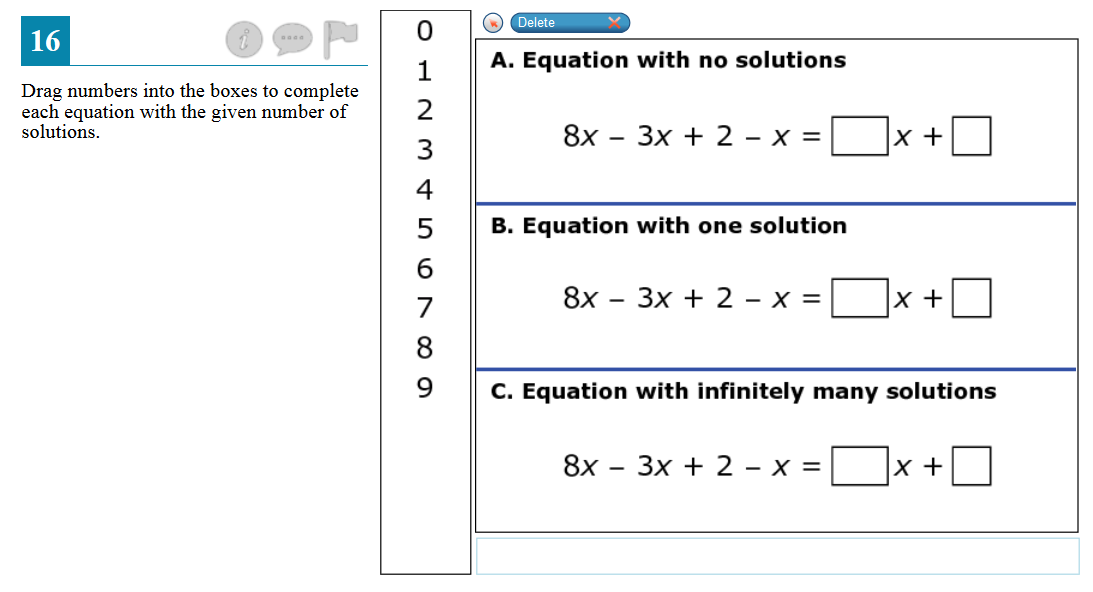Home > Grade 8 > Expressions & Equations > Solve Linear Equations with Special Cases

# Solve Linear Equations with Special Cases### Hint

What does it mean to have no solution/infinitely many solutions?  How can you make this problem simpler?  What number do you think should not go in the first box?  How can you prove that it should not go there?  How can you explain and prove that your answers are correct?What was the easiest problem to solve?  Which was the most difficult?  Why?

A.  The first box (coefficient of x) must be a 4, while the second box can be anything except for 2.

B.  The first box (coefficient of x) can be anything but a 4, while the second box can be anything.

C.  The first box (coefficient of x) must be a 4 AND the second box must be a  2.

Source: SBAC Practice Test 8th Grade

## Square Root Expression

Directions: Use the digits 1 to 9, at most one time each, to fill in …

1.Just a small error on Answer A: A. The first box (coefficient of x) must be a 4, while the second box can be anything except **from** 2.

“from” should be “for”

Thanks for doing such great work! I love this site!

•Thank you! I fixed it. Thank you again!

2.I believe the “4’s” in the answer should be “5’s”